Navigation: Realtest Script Language > Script Sections > Data Section > Self-Referential Items

As well as being able to refer to prior Data Section items, the formula of an item can also refer to itself, as it is being calculated.

Use of self-referential data item formulas is an advanced programming technique that you will most likely never require. But if you do, this shows how they work.

Data item formula calculation occurs in a loop from earliest to latest bar of each stock. (This is analogous to how you would set up a data series calculation in an Excel column, by typing a formula in the first cell, then doing "fill down". Excel automatically adjusts each cell reference to produce a running calculation.)

As a first example, let's calculate and store an "all-time high" series for each stock.

A typical formula for all-time high would be: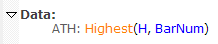This basically says "for each bar, go back through all the prior bars and find the highest high." Calculating this item this way would require 1 reference to the oldest bar, 2 references to the bar after it, 3 to the bar after that, and so on. For 10 years of data, which is about 2500 bars, calculating ATH this way would require 2500*(2500+1)/2 = 3,126,250 bar references! (Thank you, Mr. Gauss.)

Instead, if you specify the formula like this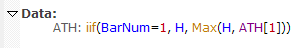then it only needs to compare two values for each bar: that bar's High, and the last calculated value of ATH. Doing it this way for 10 years of data would therefore require only 5,000 bar references, or about 1/625 as many as the first way.

Another example using this technique would be to calculate ATR using the original Welles Wilder formula as described in his book New Concepts in Technical Trading Systems. RealTest uses this same formula internally in the ATR indicator, so there's no need to calculate it yourself, but it's a fun example for this topic.

At the time that Wilder did most of his research, most traders did not have access even to a calculator, let alone a computer. He therefore favored exponential-style moving average calculation vs. using simple averages, mainly because they are so much faster to calculate by hand.

For example, ATR(14) can be calculated using this self-referential item formula: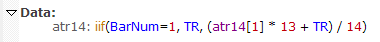To generalize this to a parameterized ATR length, you could use: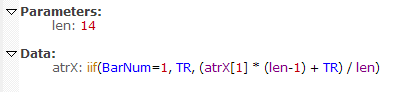Similarly, this technique can be used to calculate your own EMA, though as with ATR, there is no reason to favor this approach over simply using the EMA indicator.

For example, EMA(20) can be calculated using this self-referential item formula: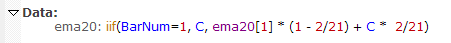To generalize this to a parameterized EMA length, you could use: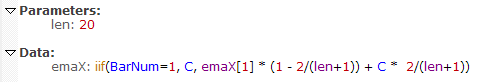You may have noticed that the EMA formula is a bit more complex than the ATR one. This may be a clue as to why Wilder preferred his non-standard smoothing technique.

For more examples of self-referential items, see flipper.rts and zigzag.rts in the Examples folder.